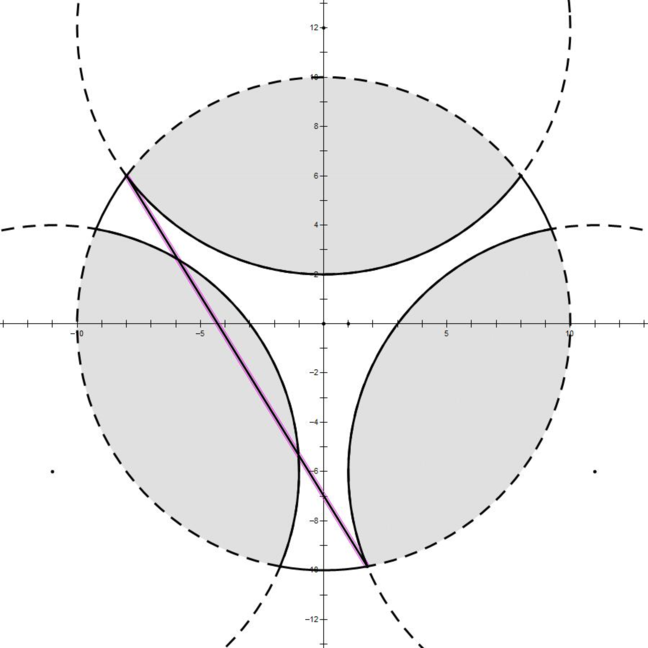# L. Machining Disc Rotors

time limit per test 8 seconds
memory limit per test 1024 megabytes
input standard input
output standard output

$Edward$ is a worker for $Aluminum$ $Cyclic$ $Machinery$. His work is to control the mechanical arms to cut out some parts of the mould material. Here is a brief introduction to his work.

Suppose the operation panel for him is a Euclidean plane with the coordinate system. Originally the mould is a disc whose centre coordinates is $(0,0)$ and of radius $R$. $Edward$ controls $n$ different mechanical arms to cut out and erase those all of the mould within their affected areas. The affected area of the $i-th$ mechanical arm is a circle whose centre coordinate is $(x_i,y_i)$ and of radius $r_i$. In order to obtain the highly developed product, it is guaranteed that the affected areas of any two mechanical arms share no intersection and no one has an affected area containing the whole original mould.

Your task is to determine the diameter of the residual mould. Here the diameter of a subset, which may not be convex, over the Euclidean plane is the supremum (i. e. the least upper bound) of distances between every two points in the subset. Here is an illustration of the sample.Input
The input contains several test cases, and the first line contains a positive integer $T$ indicating the number of test cases which is up to $5000$.

For each test case, the first line contains two integers $n$ and $R$, where $1\leq n\leq 100$ and $1\leq R\leq 1000$.

The following $n$ lines describe all mechanical arms controlled by Edward, the $i-th$ of which contains three integers $x_i,y_i$ and $r_i$ describing the affected area of the $i-th$ mechanical arm, where $−1000\leq x_i,y_i\leq 1000$ and $1\leq r_i\leq 1000.$

## Output

For each test case, output a line containing “Case #x: y” (without quotes), where $x$ is the test case number starting from $1$, and $y$ is the diameter of the remaining area with an absolute or relative error of at most $10^{−9}$. Precisely speaking, assume that your answer is $a$ and and the jury’s answer is $b$, your answer will be considered correct if $\frac{|a−b|}{max\{1,|b|\}}\leq 10^{−9}$, where $max\{x,y\}$ means the maximum of $x$ and $y$ and $|x|$ means the absolute value of $x$.

## Example

###### input
1
3 10
0 12 10
11 -6 10
-11 -6 10

###### output
Case #1: 18.611654895000252


## 题意

• 就是有一个圆心在$(0,0)$的且半径为$r$的圆$s$，另外有$n$个不相交的圆，求将$s$除去$s$与其他每一个圆的相交部分后的直径

## 题解

• 显然求出所有交点，两两枚举求$distance$取最大值即可，然后特判一下有没有一个弧是大于$180$度的，或者有某一个圆弧关于原点的对称弧与另外一个弧对称，这两种情况答案都是$2\times r$
• 然后需要注意处理好弧的方向问题，这是重点

## 代码

#include<bits/stdc++.h>

using namespace std;
#define eps 1e-9
const int maxn=505;
#define pi acos(-1.0)

int sgn(double k) {
return k<-eps?-1:(k<eps?0:1);
}

double Sqrt(double k)
{
if(sgn(k)<=0) return 0;
return sqrt(k);
}

double Acos(double a)
{
if(a>=1) return 0;
if(a<=-1) return pi;
return acos(a);
}

struct point{
double x,y;int mark;
point(double a=0,double b=0) {
x=a;y=b;
}
point operator+(point other) {
return point(x+other.x,y+other.y);
}
point operator-(point other) {
return point(x-other.x,y-other.y);
}
point operator*(double k) {
return point(x*k,y*k);
}
double operator*(point other) {
return x*other.x+y*other.y;
}
double operator^(point other) {
return x*other.y-y*other.x;
}
friend double len(point p) {
return Sqrt(p.x*p.x+p.y*p.y);
}
friend double len2(point p) {
return p.x*p.x+p.y*p.y;
}
friend double angle(point p) {
return atan2(p.y,p.x);
}

friend int point_to_line(point p,point s,point e) {
return -sgn((e-s)^(p-s));
}
friend double angle(point p1,point p2) {
if(point_to_line(p2,point(0,0),p1)<=0) return Acos((p1*p2)/len(p1)/len(p2));
else return 2*pi-Acos((p1*p2)/len(p1)/len(p2));
}
friend point extend(point p,double l) {
if(sgn(len(p))==0) return point(0,l);
return point(p*(l/len(p)));
}

friend point rotate(point p1,point p2,double a){
point vec=p2-p1;
double xx=vec.x*cos(a)+vec.y*sin(a);
double yy=vec.y*cos(a)-vec.x*sin(a);
return point(p1.x+xx,p1.y+yy);
}
};

struct circle{
point o;
double r;
circle(point a,double b) {
o=a;r=b;
}
circle(double a=0,double b=0,double c=0) {
o.x=a;o.y=b;r=c;
}
//点和圆的关系:0:圆内 1:圆上 2:圆外
friend int point_to_circle(point p,circle c) {
return 1+sgn(len(p-c.o)-c.r);
}
//圆c1与圆c2的关系:0:内含 1:内切 2:相交 3:外切 4:相离
friend int circle_to_circle(circle c1,circle c2) {
double d=len(c1.o-c2.o);
if(sgn(d-c1.r-c2.r)>0) return 4;
if(sgn(d-c1.r-c2.r)==0) return 3;
if(c1.r<c2.r) swap(c1,c2);
if(sgn(c1.r-c2.r-d)<0) return 2;
if(sgn(c1.r-c2.r-d)==0) return 1;
return 0;
}
//返回两个圆交点个数，交点坐标存在引用p1,p2中
friend int circle_circle_cross(circle c1,circle c2,point &p1,point &p2) {
int relation=circle_to_circle(c1,c2);
if(relation==0||relation==4) return 0;
double d=len(c1.o-c2.o);
if(c1.r>c2.r) swap(c1,c2);
double k=Acos((c1.r*c1.r+d*d-c2.r*c2.r)/(2*d*c1.r));
p1=c1.o+extend(rotate(c1.o,c2.o,2*pi-k)-c1.o,c1.r);
p2=c1.o+extend(rotate(c1.o,c2.o,k)-c1.o,c1.r);
if(sgn(d-c1.r-c2.r)==0||sgn(c2.r-d-c1.r)==0) return 1;
return 2;
}
};

struct node{
point s,e;
node(){}
node(point a,point b) {
s=a;e=b;
}
}o[maxn];

bool cmp(point a,point b){
return atan2(a.y,a.x)<atan2(b.y,b.x);
}

bool chec(node u,node v) {
int a=sgn(v.s^u.s),b=sgn(v.s^u.e);
int c=sgn(v.e^u.s),d=sgn(v.e^u.e);
return (a<=0&&b>=0)||(c<=0&&d>=0);
}

point p[maxn];
circle c[maxn];
int n,tot,mark[maxn],num;
double r;

int main()
{
int t;scanf("%d",&t);
for(int cas=1;cas<=t;cas++) {
tot=num=0;
scanf("%d %lf",&n,&r);
for(int i=1;i<=n;i++) scanf("%lf %lf %lf",&c[i].o.x,&c[i].o.y,&c[i].r);
circle check=circle(0,0,r);
int tot=0;
point p1,p2;
double ans=0;
for(int i=1;i<=n;i++) {
int num=circle_circle_cross(check,c[i],p1,p2);
if(num==2) {
p[++tot]=p1;
p[++tot]=p2;
point mid=rotate(point(0,0),p2,angle(p1,p2)/2);
if(point_to_circle(mid,c[i])<=1) {
p[tot-1].mark=1;
p[tot].mark=-1;
}else {
swap(p[tot],p[tot-1]);
p[tot-1].mark=1;
p[tot].mark=-1;
}
}
}
sort(p+1,p+tot+1,cmp);
for(int i=1;i<=tot;i++) for(int j=i+1;j<=tot;j++) ans=max(ans,len(p[j]-p[i]));
for(int i=1;i<=tot;i++) if(p[i].mark==-1) o[++num]=node(p[i],p[i%tot+1]);
for(int i=1;i<=num;i++) if(sgn(fabs(angle(o[i].s,o[i].e))-pi)>=0) {ans=2*r;break;}
for(int i=1;i<=num;i++) for(int j=i+1;j<=num;j++) {
node dui=node(o[i].s*-1,o[i].e*-1);
if(chec(dui,o[j])||chec(o[j],dui)) {ans=2*r;break;}
}
printf("Case #%d: %.15lf\n",cas,ans);
}
}©️2019 CSDN 皮肤主题: 技术黑板 设计师: CSDN官方博客